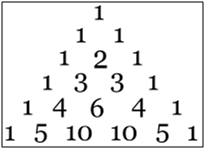# Binomial Coefficient

Posted in Statistics, Total Reads: 1600

## Definition: Binomial Coefficient

Binomial coefficients, denoted as nCr , is the number of ways in which ‘r’ items can be chosen from a set of ‘n’ items. They are a collection of positive integers that occur as coefficients in the binomial theorem. It is the number of ways of picking ‘r’ unordered outcomes from ‘n’ possibilities.

Its value is given by:-

nCr =n!/r!(n-r)!           0≤k≤n

The binomial coefficient can be arranged to form Pascal’s triangle. It is obtained by arranging binomial coefficients into rows for successive values of n, and in which r ranges from 0 to n.Hence, this concludes the definition of Binomial Coefficient along with its overview.

Browse the definition and meaning of more terms similar to Binomial Coefficient. The Management Dictionary covers over 7000 business concepts from 6 categories. This definition and concept has been researched & authored by our Business Concepts Team members.

Search & Explore : Management Dictionary

Similar Definitions from same Category: